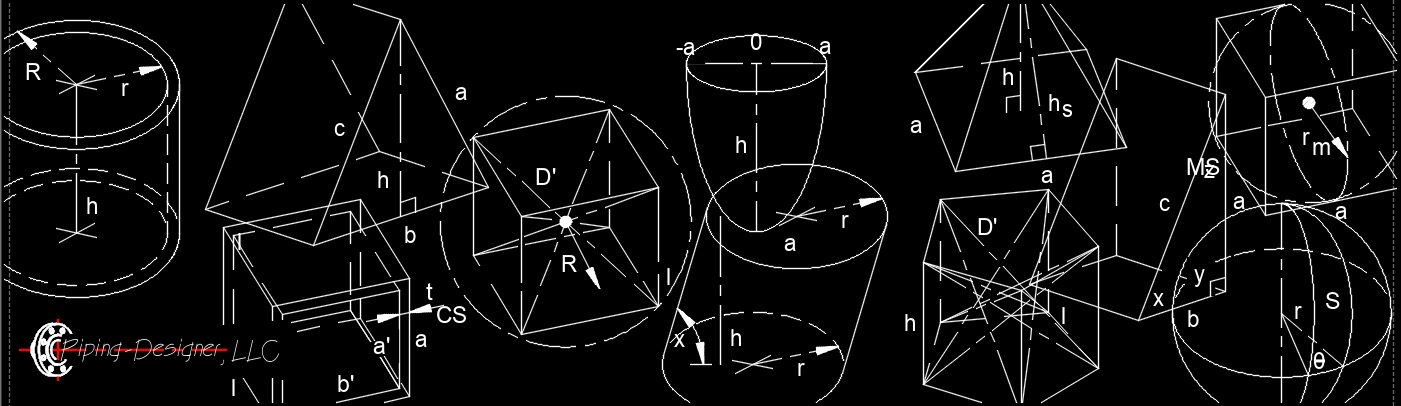## Solid GeometrySolid geometry is a three-dimensional figure with connecting edges on multiple planes.  The surface of each two-dimensional plane is called a face.

## solid Geometry Types

• Polyhedron  -  Is a solid with no curved edges or sides.
• Prism Solids  -  Prisms are solids with two congruent parallel polygon ends that are exactly the same.
• Oblique prism  -  Has with both bases not alligned above each other and the center not at 90° to the other base center.
• Right prism  -  Has both bases directly above each other and having the centers at 90° to each others base.
• Cube  -  Is a regular polyhedron with square faces.
• Right Hexagonal Prism  -  Has hexagonal bases and each face is a regular polygon with equal sides and equal angles.
• Right Octagonal prism  -
• Right Pentagonal Prism  -  Has pentagonal bases and each face is a regular polygon with equal sides and equal angles.
• Right Rectangular Prism  -  Has six faces that are rectangles with equal sides and equal angles.
• Right Square Prism  -  Has square bases, four faces that are rectangles with equal sides and equal angles.
• Right Triangular prism  -
• Pyramid Solids  -  Pyramids are solids with one polygon base and lateral faces that taper to an apex.
• Oblique Pyramid  -  The apex is not alligned above the center at 90° to the base.
• Right Pyramid  -  The apex is directly above the center at 90° to the base.
• Right Pentagonal Pyramid  -  Has a pentagon base and the apex alligned above the center of the base.
• Right Square Pyramid  -  Has a square base and the apex alligned above the center of the base.
• Right Triangular Pyramid  -  Has a triangle base and the apex alligned above the center of the base.
• Wedge Solids  -  Wedges have two triangle ends and three polygon sides.
• Non-polyhedron  -  Is a solid with curved edges or sides.
• Cone  -  Cones have one circular base tapering to an apex.
• Oblique Cone  -  The apex is not alligned above the center at 90° to the base.
• Right Cone  -  Has a circle base with the apex alligned at 90° above the center of the base.
• Cylinder  -  Cylinders have two circular parallel congruent bases.
• Oblique Cylinder  -  Both bases not alligned above each other and the center not at 90° to the other base center.
• Right Cylinder  -  Both bases direictly above each other and having the centers at 90° to each others base.
• Right Elliptic Cylinder  -  No parallel lines and both bases are ellipses.
• Right Hollow Cylinder  -  A hollow core with both bases direictly above each other and having the center at 90° to each others base.
• Ellipsoid  -  Ellipsoids have a spherical like shape with an ellipse cross section.
• Paraboloid  -
• Sphere  -  All points equally spaces from a given point of a three dimensional solid.
• Torus  -  Is shaped like a donut.

Display #
Title
Area Cross-section
Cube
Elliptic Paraboloid
Isosceles Triangle Wedge
Oblique Cylinder
Right Cone
Right Cylinder
Right Elliptic Cylinder
Right Hexagonal Prism
Right Hollow Cylinder